# Something interesting, old thread - page 14156521

...

vito333

You are rightBut, luckily, it is benign since it affects only the rsiPeriod oldest bars (the ones at the far left on the chart - with default values it is the oldest 14 bars, the rest are OK, so don't be surprised that there will be no difference at all in the rest of the bars). Anyway, updated the indicators at the post where they were posted originally (at this post : https://www.mql5.com/en/forum/181297/page9 )

Thanksvito333:

is small mistake in the "Ma Rsi Adaptive" source?

double tprice = iMA(NULL,0,1,0,MODE_SMA,price,i);

if (r<rsiPeriod)

workMaRsi[r] = price; // i suggest "tprice", not "price"?

else workMaRsi[r] =706

Nonlagmacd

Another macd this time using the nonlagma.

doc

p.s.

both files must be in indicators folder to macd works

Files:706

T3 macd

Macd of t3

doc

Files:
t3_macd.png 65 kb
t3.mq5 6 kb459

Hilbert-Huang transform and empirical mode decomposition Знакомство с методом эмпирической модовой декомпозиции - Статьи по MQL5 by the look of the article the author did not take Ehlers work as reference.I have read that in some where that the Hilbert-Huang transform is not suitable due to the cubic spline and the end effects I dont know if the Russian author brought something new for these problems in the article.156521

T3 that can be aplied to on chart indicators ...

Doc

Since know that this is what is interesting you per moment, here is a n example how an indicator that can use previous indicators data for calculation is written. It is a "good old" T3 with which you can do that. Here are examples on chart (those are applied to each other, only the first is using the regular price) and when they are applied to sub-window indicators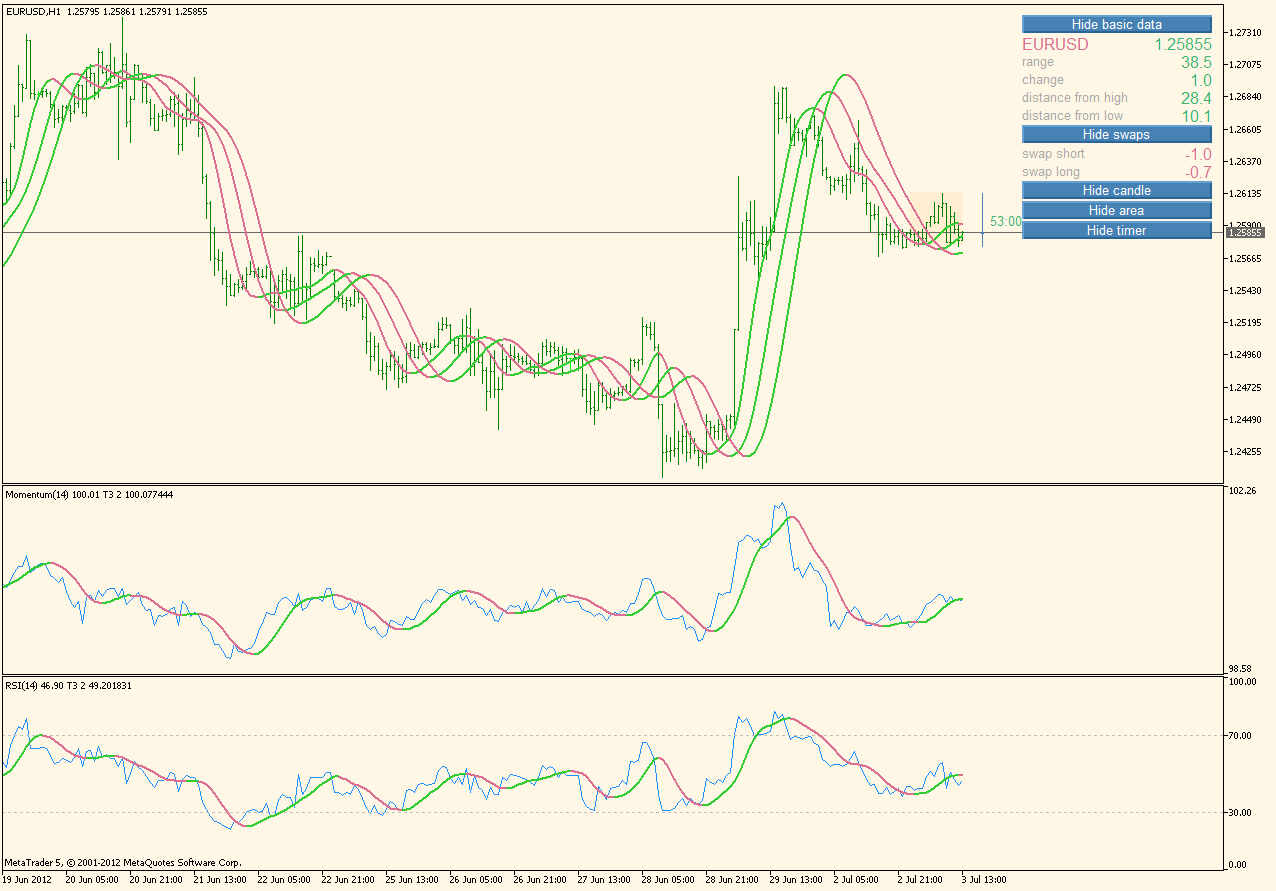Files:
5_t3.png 77 kb
t3_2.mq5 5 kb706

but how you code together rsi and (for example) sma? I tried without success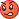You need to include the MovingAverages.mqh and called simplema function but how could I get the rsi data for the sma calculation?

p.s.

could you please explain a bit this (array range array resize??? when I need them?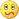):

if (ArrayRange(workT3,0) != bars) ArrayResize(workT3,bars);

if (ArrayRange(workT3Coeffs,0) < (instanceNo+1)) ArrayResize(workT3Coeffs,instanceNo+1);

Doc

Since know that this is what is interesting you per moment, here is a n example how an indicator that can use previous indicators data for calculation is written. It is a "good old" T3 with which you can do that. Here are examples on chart (those are applied to each other, only the first is using the regular price) and when they are applied to sub-window indicators898

Doc

Since know that this is what is interesting you per moment, here is a n example how an indicator that can use previous indicators data for calculation is written. It is a "good old" T3 with which you can do that. Here are examples on chart (those are applied to each other, only the first is using the regular price) and when they are applied to sub-window indicatorscouldn't understand fully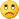b'coz don't know about coding but seems really interesting...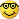Thanks156521

...

Drag it to some other indicator and in the "parameters->apply to" chose "previous indicator data" or "first indicator data"

That was not possible in metatrader 4. So, now in that example, you have there a T3 of a momentum, a T3 of a RSI, a T3 of a T3, a T3 of a T3 of a T3, all with a same indicator without changing a single line of code

secretcode:
couldn't understand fullyb'coz don't know about coding but seems really interesting...Thanks898

Drag it to some other indicator and in the "parameters->apply to" chose "previous indicator data" or "first indicator data" That was not possible in metatrader 4. So, now in that example, you have there a T3 of a momentum, a T3 of a RSI, a T3 of a T3, a T3 of a T3 of a T3, all with a same indicator without changing a single line of code:):)

You the best !156521

...

Doc

I prefer using arrays instead of buffers in calculations and then the array resize is needed.

In fact, using default settings for buffers and arrays, the only difference between arrays and buffers in metatrader 5 is that buffers are automatically adjusted their size (when the number of bars displayed on chart changes) while arrays have to be managed "manually" (the ArrayResize()) function from that code part you refer to

Also, if it was a single dimension array (liker double array[]; instead of double array[][n];) you could use the ArraySize() function instead of ArrayRange(). ArrayRange() must be used on a "non-single dimension" arrays to find out what is the effective number of array elements for a single dimension

dr.house7:

but how you code together rsi and (for example) sma? I tried without successYou need to include the MovingAverages.mqh and called simplema function but how could I get the rsi data for the sma calculation?

p.s.

could you please explain a bit this (array range array resize??? when I need them?):

if (ArrayRange(workT3,0) != bars) ArrayResize(workT3,bars);

if (ArrayRange(workT3Coeffs,0) < (instanceNo+1)) ArrayResize(workT3Coeffs,instanceNo+1);